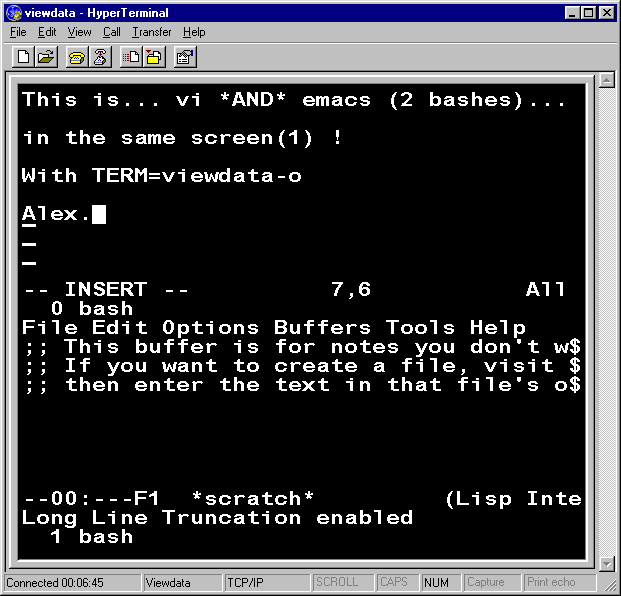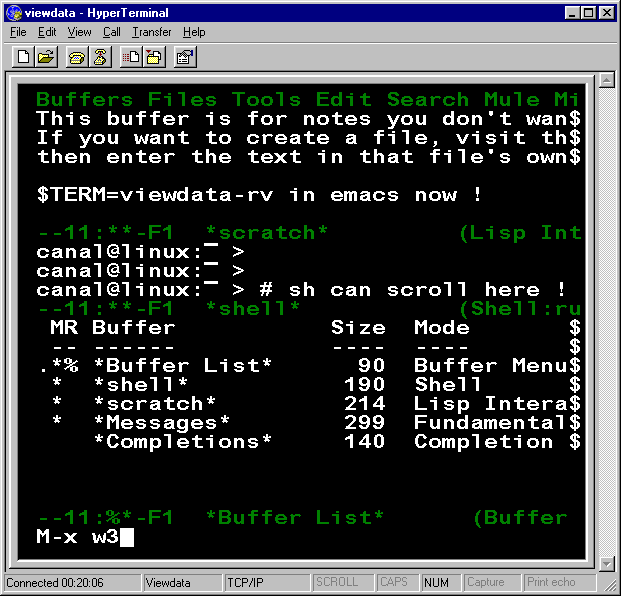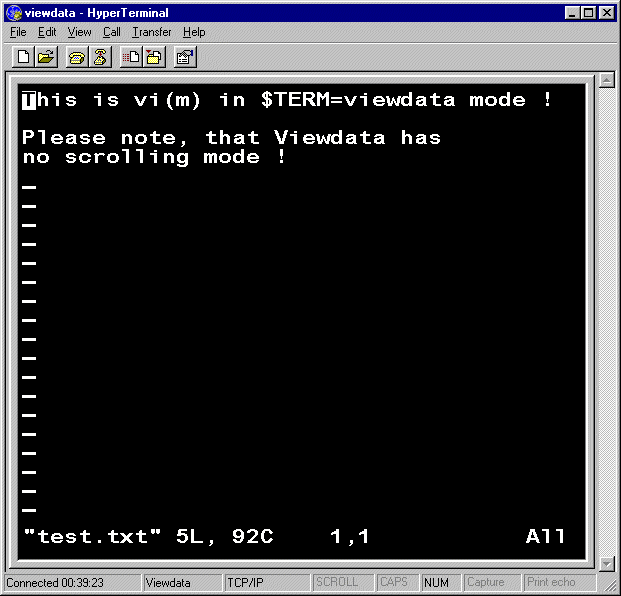RetroChallenge 2K16/01

31th Jan. : Last Day - Try it yourself !

On Linux (or Unix) with root user if possible (it works with non-root users too) :
# wget http://canal.chez.com/mntl.ti
...
# tic mntl.ti
# export TERM=viewdata-rv (or TERM=viewdata-o)
Then run emacs or screen and... Enjoy !

28th Jan. : Snapshoot for Challenge #3.

Snapshoot with TERM=viewdata-o and screen(1): vi *and* Emacs on the same Viewdata terminal :So, it's the end... ?

23rd Jan. : News and answers to Mark.

Sorry, Mark no cam(recorder) here but... I added a blinking cursor on the picture below :-D

Also to answer you, I tried on a Minitel (same codes as Viewdata for home and cursor up/down/left/right) at 1200 and 4800 Bauds. It works fine but solution #1 is a bit slow at end (bottom) of screen due to non-optimisation.
Solution #3 is far better (faster) !

24th Jan. : 2nd Optimization for Challenge #3.

viewdata-o|optimized version of viewdata prestel/viewdata terminals,
cup=^^
%p1%?%p2%{20}%>%t%?%p1%{23}%=%t%Pa%{1}%e%{1}%+%;%;%Pa

%?%ga%{13}%<%t
%?%ga%{07}%>%t^J^J^J^J^J^J^J^J%;
%?%ga%{4}%&%t^J^J^J^J%;
%?%ga%{2}%&%t^J^J%;
%?%ga%{1}%&%t^J%;
%e
%{24}%ga%-%Pa
%?%ga%{07}%>%t^K^K^K^K^K^K^K^K%;
%?%ga%{4}%&%t^K^K^K^K%;
%?%ga%{2}%&%t^K^K%;
%?%ga%{1}%&%t^K%;
%;

%?%p2%{21}%<%t
%?%p2%{07}%>%t^I^I^I^I^I^I^I^I%;
%?%p2%{15}%>%t^I^I^I^I^I^I^I^I%;
%?%p2%{4}%&%t^I^I^I^I%;
%?%p2%{2}%&%t^I^I%;
%?%p2%{1}%&%t^I%;
%e
%{40}%p2%-%Pa
%?%ga%{07}%>%t^H^H^H^H^H^H^H^H%;
%?%ga%{15}%>%t^H^H^H^H^H^H^H^H%;
%?%ga%{4}%&%t^H^H^H^H%;
%?%ga%{2}%&%t^H^H%;
%?%ga%{1}%&%t^H%;
%?%p1%{23}%=%t^K%;
%;,
use=viewdata,
This version use a maximum of 1+12+20=33 chars instead of 24+39=63 for Challenge #1 to put the cursor anywhere on the 24x40 screen. Minimum is 1 char. Average is 1+6+10=17 chars.

Average counterpart for VT100 ( ESC [ x ; y H ) is only 6 chars with a min and max of respectively 3 and 8.

And now...?

12th Jan. : Solution for Challenge #2.

viewdata-rv|prestel/viewdata terminals with reverse capabilitie (as green),
xmc#1, smso=\EB, rmso=\EG, use=viewdata,
• This "no scrolling" terminal may fool vi(m) so I'll use emacs instead.
Sample snapshoot with Hyper Terminal in viewdata-rv mode :So, next ?

7th Jan. : Solution for Challenge #1.

viewdata|prestel/viewdata terminals,
cols#40, lines#24, am, bw,
bel=^G, cr=^M,
civis=^T, cnorm=^Q,
cub1=^H, cuf1=^I, cud1=^J, cuu1=^K, home=^^, nel=^M^J,
clear=^L, .el=^X,
.ind=^J, .ri=^K,
.rep=%p1%c^R%p2%'?'%+%c, eslok, hz,
# viewdata lacks a true cup capabilitie,
# so I achieved it with home and cud1/cuf1 sequences only !
cup=^^
%?%p1%{07}%>%t^J^J^J^J^J^J^J^J%;
%?%p1%{15}%>%t^J^J^J^J^J^J^J^J%;
%?%p1%{4}%&%t^J^J^J^J%;
%?%p1%{2}%&%t^J^J%;
%?%p1%{1}%&%t^J%;
%?%p2%{07}%>%t^I^I^I^I^I^I^I^I%;
%?%p2%{15}%>%t^I^I^I^I^I^I^I^I%;
%?%p2%{23}%>%t^I^I^I^I^I^I^I^I%;
%?%p2%{31}%>%t^I^I^I^I^I^I^I^I%;
%?%p2%{4}%&%t^I^I^I^I%;
%?%p2%{2}%&%t^I^I%;
%?%p2%{1}%&%t^I%;,
(viewdata.ti file). Sample snapshoot with Hyper Terminal in viewdata mode :So, next :

Challenge 1: Making a Linux terminfo for Viewdata (Prestel)

Please note, that this seems to has never be done even since '70s !

A problem ?

The hard part is probably due to the fact that these terminals don't have a "cup" capabilitie : This mean that there is no sequence string to send cursor wherever you want on the screen (like US l+'A' c'+'A' on a french Minitel).

Or the well known "ESC [ line ; column H" sequence for DEC VT100 ones...

Solution ?

The only solution to send cursor on line y, column x with this terminal is to first go home (1,1) then doing cursor down y times and cursor right x times ...

This solution is far away from French Minitel: cup=^_%p1%'A'%+%c%p2%'A'%+%c
and famous DEC VT100: cup=\E[%i%p1%d;%p2%dH

But terminfo hold a "if-then-else" statement in its datas description. This open to a kind of developement language (but with no goto) and finally a tricky solution !